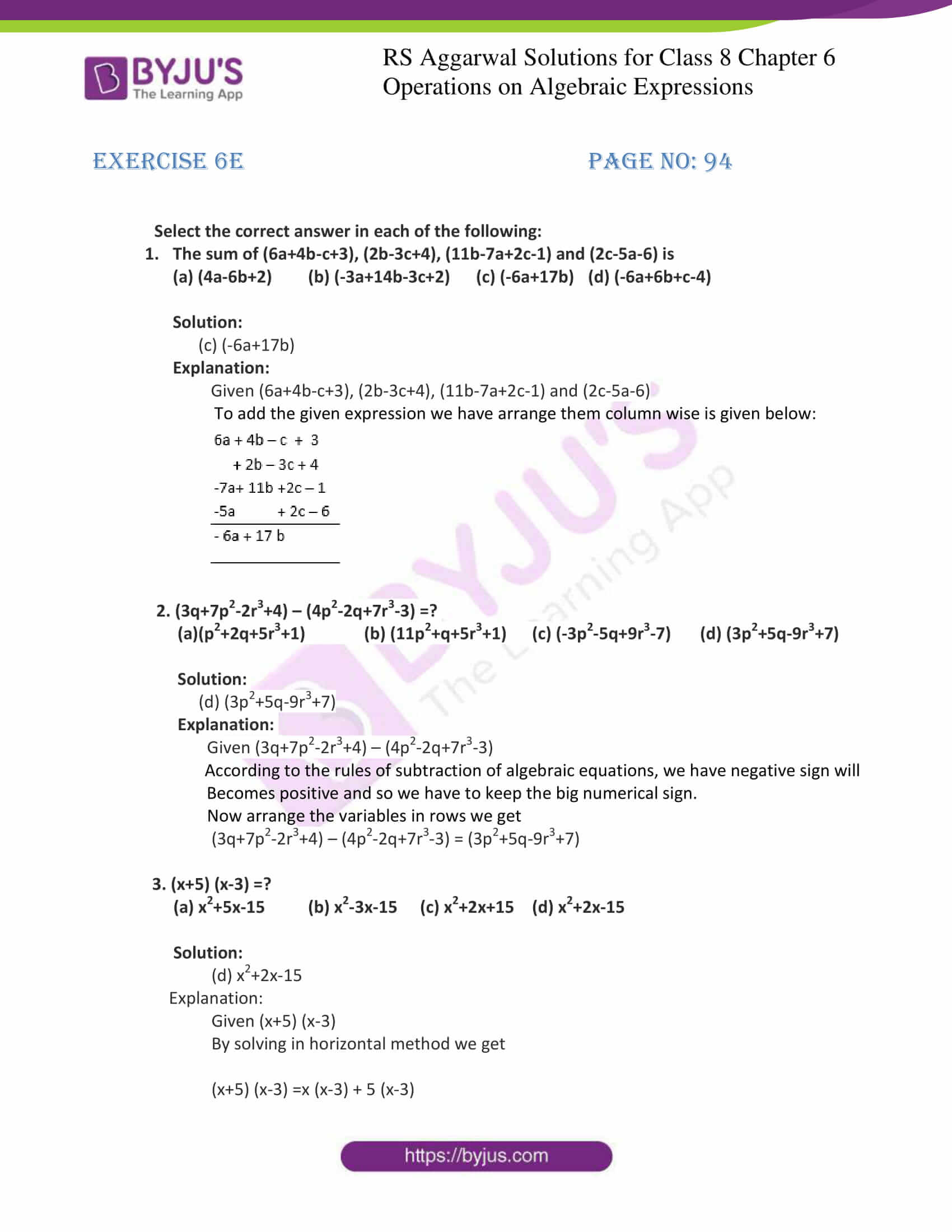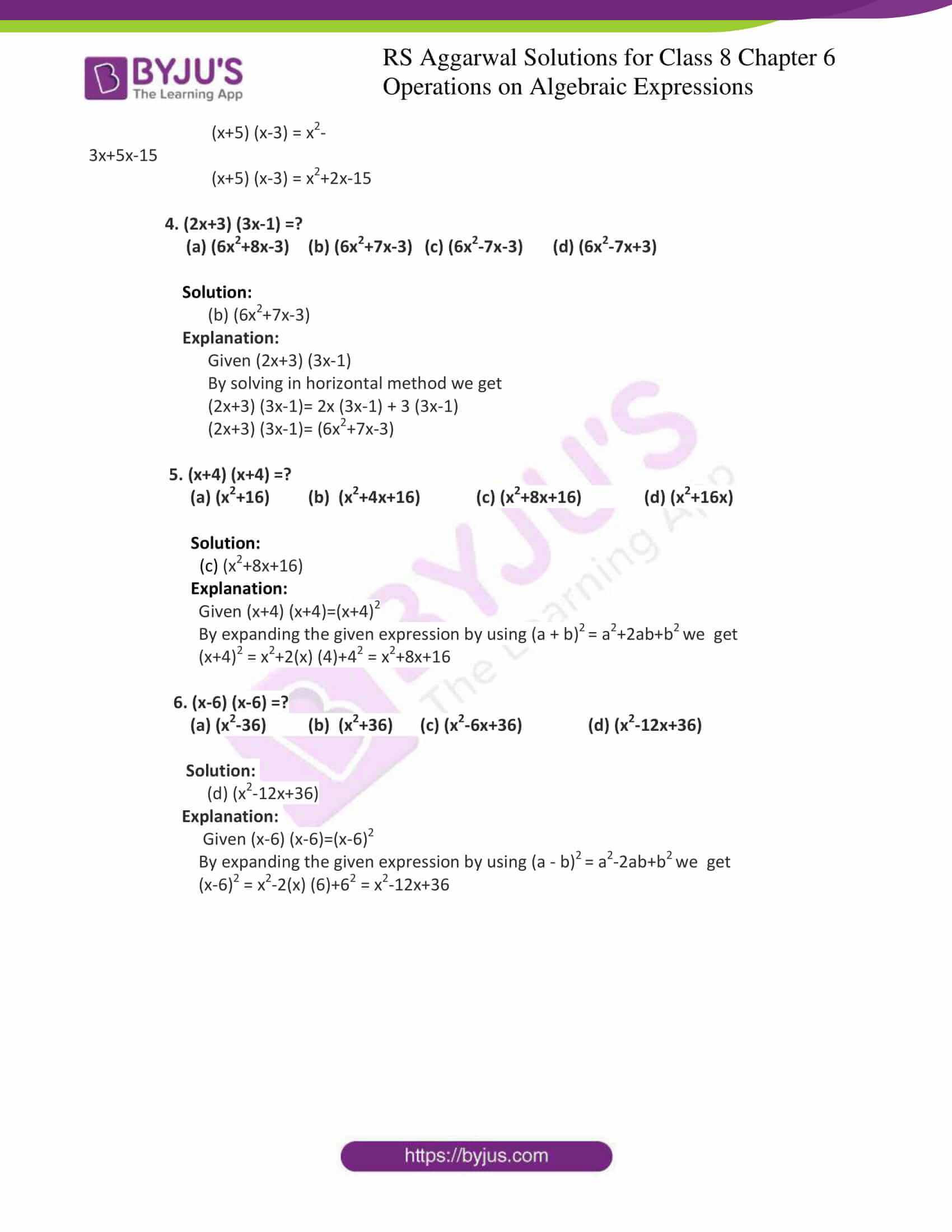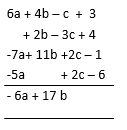# RS Aggarwal Solutions for Class 8 Maths Chapter 6 - Operations on Algebraic Expressions Exercise 6E

RS Aggarwal Solutions for the Exercise 6E of Class 8 Maths Chapter 6, Operations on Algebraic Expressions are provided here. The questions present in this exercise have been solved by BYJU’S experts in Maths, and this will help students to augment their knowledge about the topic. This exercise contains multiple-choice questions that may be used to learn and maximise time management abilities. This exercise covers all the topics included in this chapter. By practising the RS Aggarwal Solutions for class 8, students will be able to grasp the concepts perfectly.

## Download PDF of RS Aggarwal Solutions for Class 8 Maths Chapter 6 – Operations on Algebraic Expressions Exercise 6E### Access answers to Maths RS Aggarwal Solutions for Class 8 Chapter 6 – Operations on Algebraic Expressions Exercise 6E

Select the correct answer in each of the following:

1. The sum of (6a+4b-c+3), (2b-3c+4), (11b-7a+2c-1) and (2c-5a-6) is

(a) (4a-6b+2) (b) (-3a+14b-3c+2) (c) (-6a+17b) (d) (-6a+6b+c-4)

Solution:

(c) (-6a+17b)

Explanation:

Given (6a+4b-c+3), (2b-3c+4), (11b-7a+2c-1) and (2c-5a-6)

To add the given expression we have arrange them column wise is given below:2. (3q+7p2-2r3+4) – (4p2-2q+7r3-3) =?

(a)(p2+2q+5r3+1) (b) (11p2+q+5r3+1) (c) (-3p2-5q+9r3-7) (d) (3p2+5q-9r3+7)

Solution:

(d) (3p2+5q-9r3+7)

Explanation:

Given (3q+7p2-2r3+4) – (4p2-2q+7r3-3)

According to the rules of subtraction of algebraic equations, we have negative sign will

Becomes positive and so we have to keep the big numerical sign.

Now arrange the variables in rows we get

(3q+7p2-2r3+4) – (4p2-2q+7r3-3) = (3p2+5q-9r3+7)

3. (x+5) (x-3) =?

(a) x2+5x-15 (b) x2-3x-15 (c) x2+2x+15 (d) x2+2x-15

Solution:

(d) x2+2x-15

Explanation:

Given (x+5) (x-3)

By solving in horizontal method we get

(x+5) (x-3)=x (x-3) + 5 (x-3)

(x+5) (x-3)= x2-3x+5x-15

(x+5) (x-3)= x2+2x-15

4. (2x+3) (3x-1) =?

(a) (6x2+8x-3) (b) (6x2+7x-3) (c) (6x2-7x-3) (d) (6x2-7x+3)

Solution:

(b) (6x2+7x-3)

Explanation:

Given (2x+3) (3x-1)

By solving in horizontal method we get

(2x+3) (3x-1)= 2x (3x-1) + 3 (3x-1)

(2x+3) (3x-1)= (6x2+7x-3)

5. (x+4) (x+4) =?

(a) (x2+16) (b) (x2+4x+16) (c) (x2+8x+16) (d) (x2+16x)

Solution:

(c) (x2+8x+16)

Explanation:

Given (x+4) (x+4)=(x+4)2

By expanding the given expression by using (a + b)2 = a2+2ab+b2 we get

(x+4)2 = x2+2(x) (4)+42 = x2+8x+16

6. (x-6) (x-6) =?

(a) (x2-36) (b) (x2+36) (c) (x2-6x+36) (d) (x2-12x+36)

Solution:

(d) (x2-12x+36)

Explanation:

Given (x-6) (x-6)=(x-6)2

By expanding the given expression by using (a – b)2 = a2-2ab+b2 we get

(x-6)2 = x2-2(x) (6)+62 = x2-12x+36

Exercise 6A

Exercise 6B

Exercise 6C

Exercise 6D

## RS Aggarwal Solutions for Class 8 Maths Chapter 6 – Operations on Algebraic Expressions Exercise 6E

Exercise 6E of RS Aggarwal Solutions for Chapter 6, Operations on Algebraic Expressions covers all the topics related to algebraic expressions and their identities. Some of the topics focused prior to exercise 6D include the following.